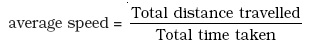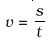Study Materials: CBSE Notes

Our CBSE Notes for Notes 8. Motion - Distance and Displacement | Class 9 Science - Toppers Study is the best material for English Medium students cbse board and other state boards students.

Notes ⇒ Class 9th ⇒ Science ⇒ 8. Motion

# Notes 8. Motion - Distance and Displacement | Class 9 Science - Toppers Study

Topper Study classes prepares CBSE Notes on practical base problems and comes out with the best result that helps the students and teachers as well as tutors and so many ecademic coaching classes that they need in practical life. Our CBSE Notes for Notes 8. Motion - Distance and Displacement | Class 9 Science - Toppers Study is the best material for English Medium students cbse board and other state boards students.

## Notes 8. Motion - Distance and Displacement | Class 9 Science - Toppers Study

CBSE board students who preparing for class 9 ncert solutions maths and Science solved exercise chapter 8. Motion available and this helps in upcoming exams 2023-2024.

### You can Find Science solution Class 9 Chapter 8. Motion

• All Chapter review quick revision notes for chapter 8. Motion Class 9
• NCERT Solutions And Textual questions Answers Class 9 Science
• Extra NCERT Book questions Answers Class 9 Science
• Importatnt key points with additional Assignment and questions bank solved.

Chapter 8 Science class 9

### Distance and Displacement class 9 Science Chapter 8. Motion

• Notes 8. Motion - Distance And Displacement | Class 9 Science - Toppers Study
• Class 9 Ncert Solutions
• Solution Chapter 8. Motion Class 9
• Solutions Class 9
• Chapter 8. Motion Distance And Displacement Class 9

## 8. Motion

(Science - IX)

Motion: when an object changes its position respect to its surrounding with time, it is said to be the object is in motion.

Example: motion of flying birds, motion of car, motion of blood in arteriesand veins etc.

Most motions are complex. Some objects may move in a straight line, others may take a circular path. Some may rotate and a few others may vibrate. There may be situations involving a combination of these.

Origin: we need to specify a reference point that describes the position of an object is called the origin.

Distance: This is total path covered by the body between its initial and final position is called distance.

Displacement: The shortest distance measured from the initial to the final position of an object is known as the displacement.

Magnitude: The numerical value of a physical quantity is its magnitude.

Physical Quantities: Quantities in physics which can be measured are called physical quantities like mass, time, area, velocity, area, density, volume, force etc.

scalars  and vectors quantities:

Scalars quantities: The quantities that require magnitudes only to specify them are called scalars quantities or scalars. e.g Mass, time, area, speed, distance, temperature, density, volume etc.

Vector quantities: Quantities that require both magnitudes and direction to specify them are called vector quantities or vectors. e.g Displacement, velocity, force, momentum, weight etc.

Differences between Displacement and Distance.

 Displacement Distance (i)  It is vector quantity. (ii) It can be negative and positive. (iii) It can be zero.  (iv) It is the shortest distance between two points. (i) It is scalar quantity. (ii) It cannot be negative. (iii) It cannot be zero. (iv) It is total path covered between to point.

Use of distance and displacement:

(i) The displacement and distance are used to describe the overall motion of an object.

(ii) They are used to locate its final position with reference to its initial position at a given time.

Motion along a straight line:

The simplest type of motion is the motion along a straight line.

When an object moves on a straight path, such a motion is called motion along a straight line or Linear motion

Examples:

1. A bus moving on a straight road.
2. A train moving on straight road.
3. A ball rolling along a straight path.

Type of Motion:

(i) Uniform Motion: As the object covers equal distance in equal intervals of time it is said to be in uniform motion.

Example:

1. Motion of car.
2. Flying of a bird.
3. Motion of a sparing.

(ii) Non-uniform Motion: As the object does not covers equal distance in equal intervals of time it is said to be in uniform motion.

Uniform Motion:

When an object covers equal distance in equal intervals of time, it is said that the object is in uniform motion.

e.g: See table

 Time Distance travelled by object A in m Distance travelled by object B in m 9:30 am   9:45 am   10:00 am  10:15 am  10:30 am  10:45 am  11:00 am 10 20  30 40 50 60 70 12 19 23 35 37 41 44

Object A covers equal distance in equal time interval, while object B does not cover equal distance in equal time interval. Therefore it is said that object A is in uniform motion and object B is in non-uniform motion.

Finding rate of motion:

Rate of motion is found by the speed of an object.

Speed: The distance travelled by the object in per unit time is called speed.

The SI unit of speed is metre per second. symbolically it is written as m s-1

Avarage speed: The average speed of an object is obtained by dividing the total distance travelled by the total time taken.If an object travels a distance s in time t then its speed v is,Important Study materials for classes 06, 07, 08,09,10, 11 and 12. Like CBSE Notes, Notes for Science, Notes for maths, Notes for Social Science, Notes for Accountancy, Notes for Economics, Notes for political Science, Noes for History, Notes For Bussiness Study, Physical Educations, Sample Papers, Test Papers, Mock Test Papers, Support Materials and Books.Mathematics Class - 11th

NCERT Maths book for CBSE Students.

books

## Study Materials List:

##### Notes ⇒ Class 9th ⇒ Science
1. Matter in Our Surroundings
2. Is Matter around us Pure
3. Atoms and Molecules
4. Structure of The Atom
5. The Fundamental Unit of Life
6. Tissues
7. Diversity in Living Organisms
8. Motion
9. Force and Laws of Motion
10. Gravitation
11. Work and Energy
12. Sound
13. Why Do We Fall ill
14. Natural Resources
15. Improvement in Food Resources

New Books